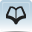# Resource 6: Problem solving - solutionsTeacher resource for planning or adapting to use with pupils

Following are some possible approaches for some example problems from Activity 3:

## Height of a tree

• Measure the length of the shadow and compare this with the length of a shadow cast by a metre rule at the same time. Use scaling to work out the height of the tree.
• Measure the length of the shadow and also the angle ϑ from the ground at the tip of the shadow to the top of the tree (Care: avoid looking at the sun!) then use
• opposite = adjacent x tan ϑ
• where opposite is the height of the tree and adjacent is the length of the shadow.

## Mass of one sheet of paper

Find the mass M of x sheets of paper, then mass of one sheet = M/x

## Area of the palm of your hand

Draw round your hand on a piece of squared paper and count the squares.

## Volume of a stone

Displacement methods (see Station 6, Extensions 1 and 2 in Resource 4)

## Thickness of a piece of paper

Measure the height (h) of a pile of x pieces of paper. One piece = h/x

## Mass of a grain of rice

Measure the mass (M) of a pile of grains. Count the grains (x). The mass of one grain = M/x.

You will need to get several people to count the grains and keep checking until everyone agrees.

## Pressure exerted by a student

A student stands on some squared paper and someone draws round their feet. The mass (M) of the same student is found in kg.

The force they exert (F) is the M x 9.8 and is in newtons. The area (A) of their feet on the ground is calculated by counting the squares.

The pressure = F/A.

Resource 5: Interesting facts about the earth

Section 3 : Pressure and heat transfer